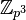# Hall polynomial

## Definition

Suppose$\mu, \nu, \lambda$ are unordered integer partitions for integers$m,n,l$ respectively such that$m + n = l$. The Hall polynomial$g_{\mu,\nu}^\lambda$ is a polynomial such that, for any prime number$p$,$g_{\mu,\nu}^\lambda(p)$ is the number of subgroups$H$ of an abelian$p$-group$G$ of type$\lambda$ such that$H$ has type$\nu$ and$G/H$ has type$\mu$.

Here, an$p$-group$P$ of type$\lambda$, where$\lambda$ is a partition into parts$a_1, a_2, \dots, a_r$, is the group$C_{p^{a_1}} \times C_{p^{a_2}} \times \dots C_{p^{a_r}}$ where$C_k$ denotes the cyclic group of order$k$.

The Hall polynomials for various triples of partitions$(\mu,\nu,\lambda)$ are related to each other. Specifically, there is an algebra, called the Hall algebra, which is a free module over the integers with generating set indexed by unordered integer partitions, and where the structure constants are the Hall polynomials.

Note that since subgroup lattice and quotient lattice of finite abelian group are isomorphic, the Hall polynomials$g_{\mu,\nu}^\lambda$ and$g_{\nu,\mu}^\lambda$ are equal.

## Particular cases

Note that since$g_{\mu,\nu}^\lambda = g_{\nu,\mu}^\lambda$ because subgroup lattice and quotient lattice of finite abelian group are isomorphic, each of our polynomials below also gives another corresponding polynomial obtained by interchanging the roles of$\mu$ and$\nu$.$\mu$$\nu$$\lambda$$g_{\mu,\nu}^\lambda(p)$ Interpretation
1 1 2$\! 1$ In a cyclic group of prime-square order$\mathbb{Z}_{p^2}$, there is a unique cyclic subgroup of prime order (isomorphic to$\mathbb{Z}_p$ such that the quotient group is also cyclic of prime order (isomorphic to$\mathbb{Z}_p$).
1 1 1 + 1$\! p + 1$ In an elementary abelian group of prime-square order$E_{p^2} \cong \mathbb{Z}_p \times \mathbb{Z}_p$, there are$p + 1$ possible subgroups isomorphic to$\mathbb{Z}_p$ where the quotient is also isomorphic to$\mathbb{Z}_p$.
2 1 3$\! 1$ In a cyclic group of prime-cube order$\mathbb{Z}_{p^3}$, there is a unique subgroup isomorphic to$\mathbb{Z}_{p^2}$ such that the quotient is isomorphic to$\mathbb{Z}_p$.
2 1 2 + 1$\! p$ In a direct product of cyclic group of prime-square order and cyclic group of prime order$\mathbb{Z}_{p^2} \times \mathbb{Z}_p$, there are$p$ many subgroups isomorphic to$\mathbb{Z}_{p^2}$ for which the quotient is isomorphic to$\mathbb{Z}_p$.
2 1 1 + 1 + 1$\! 0$ In an elementary abelian group of prime-cube order, there is no cyclic subgroup of prime-square order for which the quotient is cyclic of prime order.
1 + 1 1 3$\! 0$ In a cyclic group of prime-cube order, there is no subgroup that is elementary abelian of prime-square order for which the quotient is cyclic of prime order.
1 + 1 1 2 + 1$\! 1$ PLACEHOLDER FOR INFORMATION TO BE FILLED IN: [SHOW MORE]
1 + 1 1 1 + 1 + 1$\! p^2 + p + 1$ PLACEHOLDER FOR INFORMATION TO BE FILLED IN: [SHOW MORE]$\! m$$\! n$$\! l = m + n$$\! 1$ PLACEHOLDER FOR INFORMATION TO BE FILLED IN: [SHOW MORE]$\! 1 + 1 + \dots + 1$,$m$ times$\! 1$$\! 1 + 1 + \dots + 1$,$l = m + 1$ times$\! p^m + p^{m-1} + \dots + 1$ PLACEHOLDER FOR INFORMATION TO BE FILLED IN: [SHOW MORE]